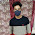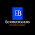# Economics Articles Library

Articles related with Micro Economics, Macro economics Accountancy and Business studies useful for XI, XII, CS foundation, CA(CPT), BBA , B.com,M.com and for other readers.

## GDP

GDP of a country means total of market value of goods and services produced in the country during an accounting year. Now we are going to understand REAL GDP and NOMINAL GDP one by one.

REAL GDP. as the nomenclature shows , Real GDP means true GDP which shows clear picture of an economy. It is also known as GDP at constant price because it is calculated on the basis of ' base year'. Base year is the year in which prices of commodities did not change substantially.Real GDP increases only when quantity increases. It adjusts all the fluctuations occured due to inflation or deflation. Real GDP is calculated as follows:-

Real GDP = quantity * price( base year)

NOMINAL GDP. does not adjust the changes in price occured due to inflation or deflation. It is calculated at current market price therefore it is called GDP at current price. In this case GDP can increase due to both factors; output and current price. It does not show clear picture of an economy.

NOMINAL GDP= Quantity * price( current)

Now we can compare with example. If in the year 2017 , cloth measured as 5000 metre and price was 50Rs then nominal GDP was 5000*50= 1,25,000. Next year in 2018, output remains same but price increased from 50Rs to 60Rs then we shall get nominal GDP as 1,30,000. What do you think now? Hope you understand. It shows only growth in monetary terms which is not actually growth of an economy.

Real GDP only increases when quantity of output increases which is actual growth of an economy. It increases social welfare.

Social welfare is defined in terms of availability of goods per person. When output increases at the rate which is more than the rate of population, availability of goods and services increases per person.

1.1.